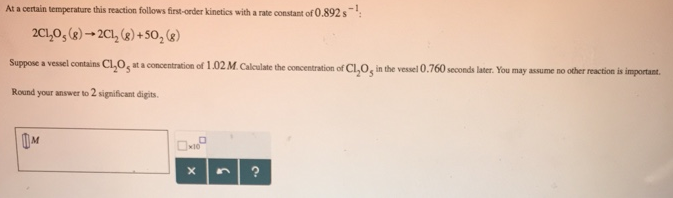# Problem: At a certain temperature this reaction follows first-order kinetics with a rate constant of 0.892s-1: 2CI2O5 (g) → 2CI2 (g) + 5O2 (g) Suppose a vessel contains CI2O5 at a concentration of 1.02 M. Calculate the concentration of CI2O5 in the vessel 0.760 seconds later. You may no other reaction is important. Round your answer to 2 significant digits.

###### FREE Expert Solution
80% (155 ratings)###### Problem Details

At a certain temperature this reaction follows first-order kinetics with a rate constant of 0.892s-1

2CI2O(g) → 2CI2 (g) + 5O2 (g)

Suppose a vessel contains CI2O5 at a concentration of 1.02 M. Calculate the concentration of CI2O5 in the vessel 0.760 seconds later. You may no other reaction is important.